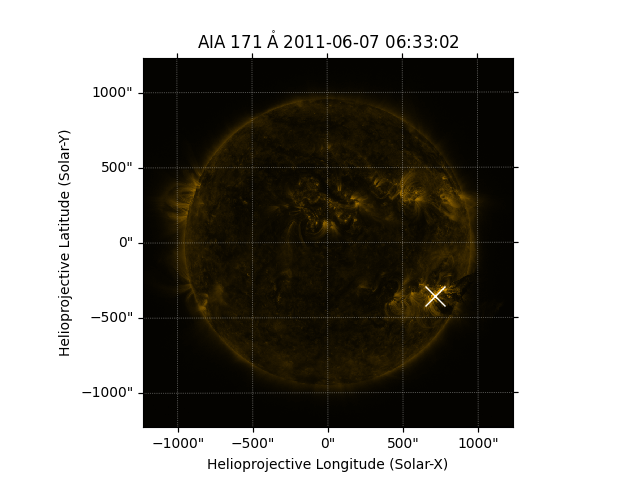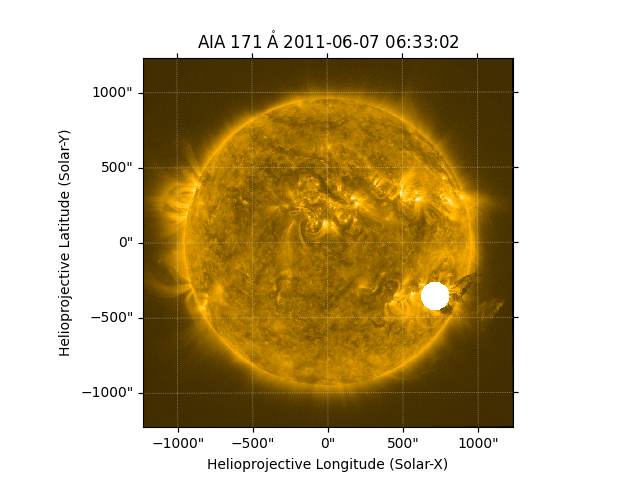# Finding and masking bright pixels¶

How to find and overplot the location of the brightest pixel and then mask any pixels out the area around this region.

from __future__ import print_function, division

import numpy as np
import numpy.ma as ma
import matplotlib.pyplot as plt

import astropy.units as u

import sunpy.map
from sunpy.data.sample import AIA_171_IMAGE


We first create the Map using the sample data and import the coordinate functionality.

aia = sunpy.map.Map(AIA_171_IMAGE)


Now to find the single brightest pixel, we will maximium of the AIA image. Note that we transform from pixel space to the world corodiate system.

pixel_pos = np.argwhere(aia.data == aia.data.max())*u.pixel
hpc_max = aia.pixel_to_world(pixel_pos[:, 1], pixel_pos[:, 0])


Let’s now plot the results. We will overlay the SunPy lon/lat grid.

fig = plt.figure()
ax = plt.subplot(projection=aia)
aia.plot()
ax.plot_coord(hpc_max, 'bx', color='white', marker='x', markersize=15)
plt.show()Now we create a new custom AIAMap with a mask around the brightest pixel. We have to build two arrays which include all of the x and y pixel indices. These indices are for the entire image. We must not forget to add the correct units because we will next pass into a SunPy function which all require them.

x, y = np.meshgrid(*[np.arange(v.value) for v in aia.dimensions]) * u.pixel


Now we can convert this to helioprojective coordinates and create a new array which contains the normalized radial position for each pixel adjusted for the position of the brightest pixel (using hpc_max).

hpc_mask = aia.pixel_to_world(x, y)
r_mask = np.sqrt((hpc_mask.Tx-hpc_max.Tx) ** 2 + (hpc_mask.Ty-hpc_max.Ty) ** 2) / aia.rsun_obs
mask = ma.masked_less_equal(r_mask, 0.1)
scaled_map = sunpy.map.Map(aia.data, aia.meta, mask=mask.mask)


Let’s now plot the new Map!

fig = plt.figure()
ax = plt.subplot(projection=scaled_map)
scaled_map.plot()
plt.show()Total running time of the script: ( 0 minutes 1.320 seconds)

Gallery generated by Sphinx-Gallery